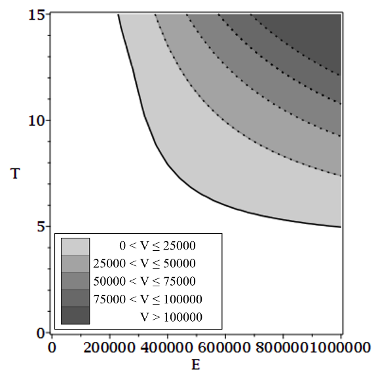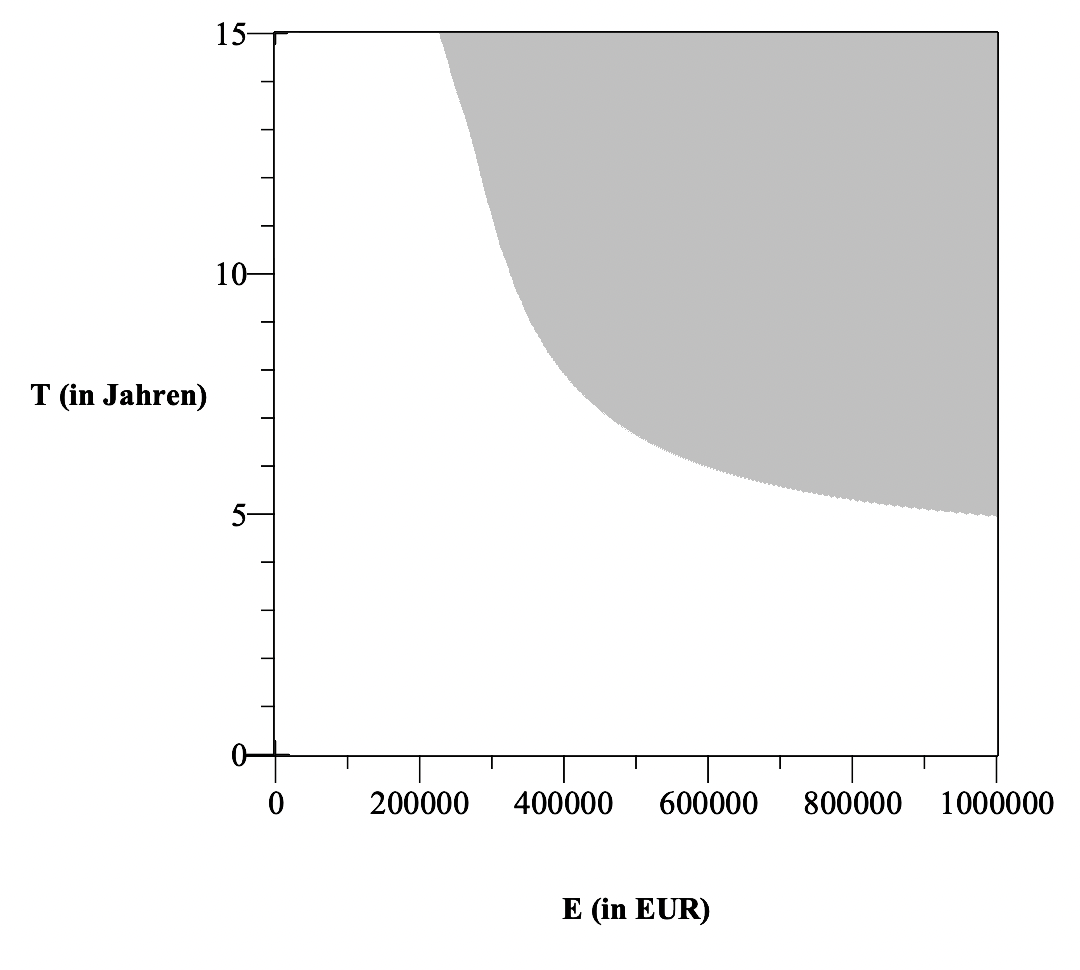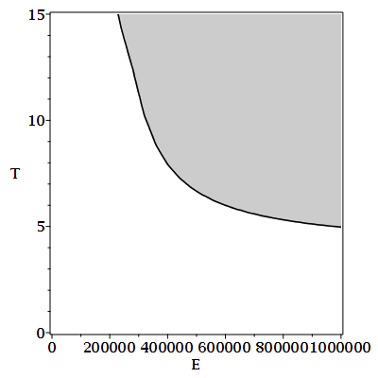2 years, 43 days

## coloring surfaces in a plot...

Maple 2021

Hello,

Im a tax student and tring to figure out Maple. I plotted the following graph with the code:

implicitplot([Vth = Vps, V1 = 25000, V1 = 50000, V1 = 75000, V1 = 100000], E = 0 .. 1000000, T = 0 .. 15, color = [black], labels = ["E in (EUR)", "T (in Jahren)"], labelfont = [times, bold, 12], view = [0 .. 1000000, 0 .. 15])I would like to color the intermediate areas and attach a legend. A colleague has plotted this for me, but unfortunately has not provided me with the source code and has now lost the file. Can someone help me to re-plot the last shown chart?Thanks so so much in advance!! Best regards Rebekka

## Draw indifference curve in 3d plot...

Maple 2021

Hello,

Im a tax student trying to use Maple for my studies. I plotted two surfaces in a 3D coordinate system, the surfaces intersect = indifference area.

The current formula is like this:

plot3d([Vth/E, Vps/E], E = 0 .. 1000000, T = 0 .. 15, labels = ["E", "T", ""], color = [gray, white], labels = ["E (in EUR)", "T (in Jahren)", ""], labelfont = [times, bold, 12])
It looks like this:I would like to draw in the same graph, the indifference area (Vth/E = Vth/E), it looks like this:A colleague has plotted this for me, but unfortunately has not provided me with the source code and has now lost the file. Can someone help me to re-plot the last shown chart?

Rebekka

## How to plot absolute advantage of option...

Maple

Dear community,

this is a related questio to "Determine zeros, indifference curve of two levels, numerical approximation".

I am  trying to model the advantage of corporate taxation (final asset value: V_TEV) over partnership taxation (final asset value: V_P).
I would like to plot the absolute advantage of corporate taxation (VT=V_TEV-V_P) as a function of income (E) and retention period (T). Here x=E, Y=T. I alread plotted the VT. However, Id like to have the graph differently:
I would like to construct the graph in such a way that the absolute wealth advantage VT can be read from the ordinate. Can someone please help me with that?

Here are the formulars, the study is based on:

E := 'E'
h := 4
m := 0.035
g:= h*m
k := 0.15
a := 0.25;
F_ANR := 4*m;
r:= 0.1;
T := 'T';
ESt := piecewise
(E < 9984, 0,
E < 14926, (1008.7*(E - 9984)/10000 + 1400)*(E - 9984)/10000,
E < 58596, (206.43*(E - 14926)/10000 + 2397)*(E - 14926)/10000 + 938.24,
E < 277826, 0.42*E - 9267.53,
0.45*E - 17602.28);

GewSt := piecewise(E < 24500, 0, (E - 24500)*g)

stev := EStk/K

K := 0.6*E*(1 - k - g)*(1 + r*(1 - k - g))^T

EStk := piecewise(K < 9984, 0,
K < 14926, (1008.7*(K - 9984)/10000 + 1400)*(K - 9984)/10000,
K < 58596, (206.43*(K - 14926)/10000 + 2397)*(K - 14926)/10000 + 938.24,
K < 277826, 0.42*K - 9267.53,
0.45*K - 17602.28)

V_TEV := E*(1 + (-k - g))*(1 + r*(1 - k - g))^T*(1 - 0.6*stev)

Er := E + (E - ESt)*r

EStr := piecewise(Er < 9984, 0,
Er < 14926, (1008.7*(Er - 9984)/10000 + 1400)*(Er - 9984)/10000,
Er < 58596, (206.43*(Er - 14926)/10000 + 2397)*(Er - 14926)/10000 + 938.24,
Er < 277826, 0.42*Er - 9267.53,
0.45*Er - 17602.28);

srp := EStr/E

r_p := r*(1 - srp)

V_P := (E - ESt + GewSt - min(ESt, GewSt, E*F_ANR))*(1 + r_p)^T

VT := V_TEV - V_P

plot3d(VT, E = 0 .. 500000, T = 1 .. 15, axes = boxed)

Thank you so much in advance!! Rebekka

## Determine zeros, indifference curve of t...

Maple

Dear community,

I'm a taxation student and trying to figure out maple more or less successfully for a research project... My aim is to find the indifference curve of the two levels S_TEV and S_P. As its probably not easy to find the formula, I'd alternatively like to approach the indifference curve numerically.

These are the basis codes:

E := 'E'
h := 4
m := 0.035
g:= h*m
k := 0.15
a := 0.25;
F_ANR := 4*m;
r:= 0.1;
T := 'T';
ESt := piecewise
(E < 9984, 0,
E < 14926, (1008.7*(E - 9984)/10000 + 1400)*(E - 9984)/10000,
E < 58596, (206.43*(E - 14926)/10000 + 2397)*(E - 14926)/10000 + 938.24,
E < 277826, 0.42*E - 9267.53,
0.45*E - 17602.28);

Er := E + (E - ESt)*r

EStr := piecewise(Er < 9984, 0,
Er < 14926, (1008.7*(Er - 9984)/10000 + 1400)*(Er - 9984)/10000,
Er < 58596, (206.43*(Er - 14926)/10000 + 2397)*(Er - 14926)/10000 + 938.24,
Er < 277826, 0.42*Er - 9267.53,
0.45*Er - 17602.28)

srp := EStr/E

r_p := r*srp

S_P := ESt*(1 + r_p)^T

EStk := piecewise(K < 9984, 0,
K < 14926, (1008.7*(K - 9984)/10000 + 1400)*(K - 9984)/10000,
K < 58596, (206.43*(K - 14926)/10000 + 2397)*(K - 14926)/10000 + 938.24,
K < 277826, 0.42*K - 9267.53,
0.45*K - 17602.28)

r_tev := 0.29*r

K := (0.71*E*(1 + 0.71*r)^T)*0.6

stev := EStk/K
S_TEV := E*(0.29*(1 + r_tev)^T + 0.71*(0.6*stev))

plot3d([S_TEV/E, S_P/E], E = 0 .. 500000, T = 0 .. 15, color = [white, black])

The plot shows the two levels. Id like to derive the indiffernce curve from this plot (intersection of S_TEV and S_PE).
Starting with probably Diff_S := S_TEV - S_P?

Can someone please help me with finding the right codes? I'm lost.... (eg. solve function for S-TEV-S_P=0; finding the right data frame for Diff_S:=0 or almost zero dependent on E and T, i.e. dataframe of E, T and S_TEV and S_P) so that I can plot (3D) all S_TEV = S_P depending on E, T.

Id be incredibly thankful for any help. It would literally safe my thesis!
Thank you so so much in advance!!
Rebekka

## How do I import only selected columns fr...

Maple

Hello community,

how can I import only selected columns from a csv file?
My current code is the following:

IK := Import("Indifferenzkurve.csv", output = datadir)

However, I'd like to only import the three columns "E","T", and "S_P and S_TEV", not column " " and "Diff".
On this basis, I'd like to plot "S_P and S_TEV":

plot3d([IK], E = 0.1 .. 1000000, T = 1 .. 45)